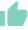# RULE OF 70Gaurav Seth
20 Likes | 423 days agoLikeShareAuthor is a Zfunds Verified ExpertGaurav Seth
Gurugram## RULE OF 70

The rule of 70 is an easy and simple mathematical formula that is used to approximate how much time it takes an investment to double down its value. It is similar to the rule of 69 and that of rule of 72, but has slightly different applications. Let’s understand the same, its meaning, working and how it is used.

## MEANING

Investors usually use the rule of 70 to predict the years it will take for their investment to double in terms of value on a specific rate of interest/return. (An investment's profit or loss over a period of time). This rule is generally used to compare investments with different annual rates of interest. This makes it easier for the investors to figure out how long it may be before they witness similar returns on their money from each of the investment alternatives.

Let’s assume an investor decides to compare returns on the investments in their retirement portfolio to get a brief idea of how long it will take the savings to double. To calculate this time of doubling, the investor will simply divide 70 by the annual rate of return.

Here is an illustration:

• At a 4% rate of growth, it will take 17.5 years for a portfolio to double its value (70/4)
• At a growth rate of 7%, it will take 10 years to double down (70/7)
• At a growth rate of 11%, it will take around 6.4 years to double (70/11)

## WORKING OF RULE OF 70!

Now that we have seen the rule of 70 and its application, let us break down the formula so you understand how to apply this rule to your own investment and portfolio.

Again, calculating the rule of 70 is pretty simple. All you have to do is divide 70 by the estimated annual rate of return to find how many years it will take the investment to double down its value. For the calculation to work fairly and properly, you will need to have at least an estimate of annual growth or returns of investment.

## NEED OF RULE OF 70

Keeping in mind that this rule is purely a rough estimate, but it can be really handy. But if you want a more concrete way of looking at the potential of a mutual fund, retirement portfolio or other investment alternatives than the interest rate alone could provide. Knowing the number of years it will take to reach a desired value can help you plan which alternative to opt for your retirement portfolio.

For instance:

Let us say you wanted to pick a precise mix of investments with the potential to grow a certain value by the time you retire in 20 years time. You could use this rule to calculate the doubling time for each investment under consideration to see if it can help you reach your savings goals by the time you are going to retire.

## MERITS

1. To use the rule of 70, you just have to do a simple division which makes it a very straightforward formula.
2. This rule makes it easy to estimate the years it will take for the value of investment to double down and hence making it a strong investment growth prediction model.

## DOWNSIDES

1. The reason this rule is not always accurate is because it assumes an investment is compounding without any break. But most financial institutions calculate the returns less frequently so this assumption is inherently flawed when it comes to the ability to predict accurate time for this rule.
2. While this rule can provide a basic calculation of how long it may take for an investment to double down, the calculation is only an estimation. Moreover, this estimate can be thrown off by fluctuation in growth and interest rates.

## ALTERNATIVE

The rule of 69 and 72 are the alternatives available. They differ in their accuracy for investments with different compounding frequencies (which measures how often the interest is compounding). Both functions are identical to the rule of 70, except they divide the annual rate of return with 72 and 69 to derive the time of doubling.

Usually, the rule of 69 is believed to be more accurate for calculating doubling time for continuously compounding intervals especially at a lower interest rate. The rule of 70 is deemed to be more accurate for semi-annual compounding while the rule of 72 is more accurate when it comes to annual compounding.